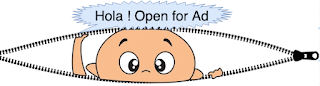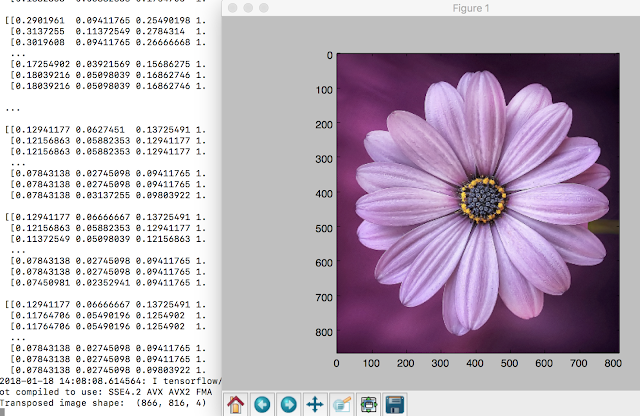## Latest Posts

A machine learning algorithm is an algorithm that is able to learn from data and apply that learning to make instructive decision. Most commonly known ML problems are : Classification, Regression, Clustering. In traditional ML based problems input to the system(feature vectors) are decided by experts(knowledgeable entity) and it requires sound judgement.

Consider a system which figure out by themselves what features to pay attention and mark for feature vectors is termed as “Representation” ML-based systems. Deep learning systems are one type of representation systems. In other words, Deep Learning Algorithms learn what features(characteristics/attributes) requires more attention and each features are represented by data points (Vector). Neural Networks is most common and de facto class of deep learning algorithms.  Neural networks help find unknown patterns in massive data sets. "Neurons" are most basic building blocks that actually “learn”. Below image shows high level overview where does deep learning algorithm fits.Deep Learning(Layers of inter connected neural networks) based Binary Classifier(Source: PluralSight)
What is relation between Machine learning and Deep learning ?
Deep learning is subfield/subset of Machine learning. Machine learning blossoms Artificial intelligence with bang. However, Deep learning giving birth of limitless growth of AI. The very way to visualise how Deep learning influenced AI development in big way - Google Deep learning program ApphaGo beat GO Master Lee Sedol.

What is TensorFlow and TensorBoard -  Deep learning de facto library and dashboard
Official doc of TensorFlow states it is an open source software library for numerical computation using data flow graphs. Everything in TensoFlow is a graph - nodes of graph represents operations and data flowing through graph and transformed along the way is termed as "Tensors".

Tensors : Central Unit of data in TensorFlow. A tensor consists of a set of primitive values shaped into an array of any number of dimensions. i.e : [1,2,3] , [[1,2],[2,3]], etc.  Scalers(2, 3.5, etc) are 0-D tensor, vector [2,4,6] are 1-D tensors, matrix are 3-D tensors.
Rank, shape and data types are 3 important characteristics which define a Tensor. The number of dimensions in a tensor is Rank of tensor. and the number of elements in each dimension Shape of tensor.
Tensors Rank
4 0
[1,2,3] 1
[[1,3],[4,6]] 2
[[,,,]] 4

Tensors Shape
4 []
[1,2,3] 
[[1,4,3],[6,8,9]] [3,2]
[[,,,]] [2,2,1]
What makes TensorFlow so special and why it's on the way to become default library for machine learning ?
• TensorFlow provides a foundation for development of new ML algorithms and it supports large scale distributed models from training to production.
• It provides stable, efficient and performant python API.
• TensorFlow goes hand in hand with TensorBoard which gives graphical view(in the form of dashboard) of graph/activity with minute details for analysis.
TensorBord is a suite of visualisation tool which we can use to visualise your TensorFlow graph, plot quantitative metrics about the execution of your graph, and show additional data like images that pass through it.

Refer TensorFlow official guide to Install and setup TensorFlow environment. Once tensorFlow is setup prompt is changed and open python prompt, execute some commands(follow below lines of commands) to test installation.
```➜  ~ source /Users/n0r0082/TensorFlowWork/bin/activate
(TensorFlowWork) ➜  ~ python
Python 2.7.10 (default, Jul 15 2017, 17:16:57)
[GCC 4.2.1 Compatible Apple LLVM 9.0.0 (clang-900.0.31)] on darwin
>>> import tensorflow as tf
>>> hello = tf.constant('Hello, TensorFlow')
>>> sess = tf.Session()
2018-01-16 23:26:11.178955: I tensorflow/core/platform/cpu_feature_guard.cc:137] Your CPU supports instructions that this TensorFlow binary was not compiled to use: SSE4.2 AVX AVX2 FMA
>>> print(sess.run(hello))
Hello, TensorFlow
```

In order to explore usefulness of TensorBoard, we will perform some mathematical operations(square ,power, square root and addition) using python tensorFlow API's and construct graphs out of these operation & visualise the same in tensorBoard.
```import tensorflow as tf

#create constants
a = tf.constant(6.5,name='constant_a')
b = tf.constant(3.4,name='constant_b')
c = tf.constant(3.0,name='constant_c')
d = tf.constant(100.2,name='constant_d')

#compute square, power and Sqrt
square = tf.square(a,name="square_a")
power = tf.pow(b,c,name="power_b_c")
sqrt =tf.sqrt(d,name="sqrt_d")

#create session
sess = tf.Session()

#Execute above operations using session object
print "square is ", sess.run(square)
print "power is ", sess.run(power)
print "sqrt is ", sess.run(sqrt)
print "total sum is ", sess.run(final_sum)

# Create writer object for tensorBoard and save operations as grapgh at ./m2_eample
writer = tf.summary.FileWriter('./tensorBoardDir',sess.graph)
#close resources used.
writer.close()
sess.close()
```

Execute above sample and find results
```(TensorFlowWork) ➜  samples python sample-2.py
2018-01-17 15:08:16.781469: I tensorflow/core/platform/cpu_feature_guard.cc:137] Your CPU supports instructions that this TensorFlow binary was not compiled to use: SSE4.2 AVX AVX2 FMA
square is  42.25
power is  39.304005
sqrt is  10.0099945
total sum is  91.563995
```

Using following command we can start tensorBoard which launches dashboard On some URL.
```(TensorFlowWork) ➜  ~ tensorboard --logdir="tensorBoardDir"
TensorBoard 0.4.0rc3 at http://m-C02T75XBG8WN:6006 (Press CTRL+C to quit)
```

Launch dashboard in browser at "http://0.0.0.0:6006". Below screenshot shows each operation as node and data flow from one operation to another as Tensor.

Each node represents operation and output of these three operations are tensors- feed into another operations final_sum.

Image recognition and processing with TensorFlow: Image is collection of pixel and each pixel contains some value. Coloured image is represented in the form of RGB values(3 channels) for each pixel and greyscale image each pixel stores value of intensity between 0.0 - 1.0 (1 channel). Here we will read an image with TensorFlow library and store image as tensors followed by rotate image - find transpose of image.
```import tensorflow as tf
import matplotlib.image as mp_img
import matplotlib.pyplot as plot
import os

#input file
filename = "./input.png"

#get image object from input file

print "Image shape is: ", image.shape
print "Image array is: ", image

#plot.imshow(image)
#plot.show()

#create a variablefor holding image data
x = tf.Variable(image, name='x')

#inititlize all global variables
init = tf.global_variables_initializer()

with tf.Session() as sess:
sess.run(init)
#compute transpose of variable x (image matrix data)
transpose = tf.image.transpose_image(x)
#Run transpose data.
result = sess.run(transpose)
# Display shape of image - first two values shoudld be swapped.
print "Transposed image shape: ", result.shape
plot.imshow(result)
plot.show()
```

Sample output: -
```(TensorFlowWork) ➜  samples python sample-5.py
Image shape is:  (816, 866, 4)
Image array is:  [[[0.30980393 0.10980392 0.27058825 1.        ]
[0.30588236 0.10196079 0.27058825 1.        ]
[0.31764707 0.10980392 0.28235295 1.        ]
...
[0.18431373 0.05490196 0.17254902 1.        ]
[0.18039216 0.04705882 0.16470589 1.        ]
[0.16862746 0.03921569 0.15686275 1.        ]]

[[0.29803923 0.10196079 0.2627451  1.        ]
[0.30588236 0.10588235 0.27058825 1.        ]
[0.32156864 0.11372549 0.28627452 1.        ]
...
[0.18039216 0.05098039 0.16862746 1.        ]
[0.17254902 0.03921569 0.15686275 1.        ]
[0.1882353  0.05882353 0.1764706  1.        ]]
...
[0.07843138 0.02745098 0.09411765 1.        ]
[0.07843138 0.02745098 0.09411765 1.        ]
[0.07843138 0.02745098 0.09803922 1.        ]]]

Transposed image shape:  (866, 816, 4)
```

Image orientation comparison :- First image is original image(un-comment line #15 and 16 to generate it) and second image is transposed image.

Original image with shape (816,866,4) and channel value is 4.Original image displayed via tensorFlowTransposed image displayed via tensorFlow
Transposed Image (Rotated by 90*) with shape (866,816,4) and channel value is 4. If we assume original axis index are 0,1,2 on transpose first two axis are swapped.

Note:- Using TensorFlow library we can re-size images, flip images too.

===== ****** =====

1.2.The development of artificial intelligence (AI) has propelled more programming architects, information scientists, and different experts to investigate the plausibility of a vocation in machine learning. Notwithstanding, a few newcomers will in general spotlight a lot on hypothesis and insufficient on commonsense application. machine learning projects for final year In case you will succeed, you have to begin building machine learning projects in the near future.

Projects assist you with improving your applied ML skills rapidly while allowing you to investigate an intriguing point. Furthermore, you can include projects into your portfolio, making it simpler to get a vocation, discover cool profession openings, and Final Year Project Centers in Chennai even arrange a more significant compensation.

Data analytics is the study of dissecting crude data so as to make decisions about that data. Data analytics advances and procedures are generally utilized in business ventures to empower associations to settle on progressively Python Training in Chennai educated business choices. In the present worldwide commercial center, it isn't sufficient to assemble data and do the math; you should realize how to apply that data to genuine situations such that will affect conduct. In the program you will initially gain proficiency with the specialized skills, including R and Python dialects most usually utilized in data analytics programming and usage; Python Training in Chennai at that point center around the commonsense application, in view of genuine business issues in a scope of industry segments, for example, wellbeing, promoting and account.

3.4.I like your post. I appreciate your blogs because they are really good. Please go to this website for Data analyst course in Bangalore. These courses are wonderful for professionals.